# Propositional LogicExamples

The sentential logic of Principia Metaphysica is classical. Although we have presented the logic axiomatically, our axiom system has the same power as the `natural deduction' systems of sentential logic that you find in any introductory text. These natural deduction systems present the logic by describing introduction and elimination rules for the connectives. These rules tell one how to draw inferences to and from sentences involving these connectives within a proof.

However, since we are using the axiomatic method rather than a natural-deduction system, we first present the logic axiomatically and then prove that the introduction and elimination rules used in `natural deduction' systems are valid rules. The axiomatic development of our sentential logic is presented in the item Logic/Sentential Logic. For convenience, we reproduce the axioms and rules of sentential logic here: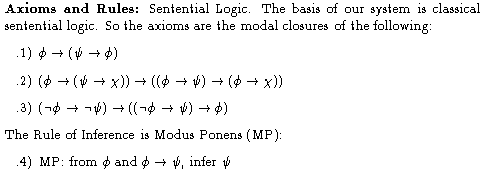In what follows, we draw out consequences and prove some facts about this logic.

## Some Consequences:

In this section, we offer some basic examples of how to prove consequences of the logical axioms using the rules of inference. Basically, the method is to produce proof sequences. With this method, all of the tautologies of sentential logic can be derived as logical theorems.

Theorem of the Theory p:This asserts that from the single premise p we can derive the conditional if q then p. To see that this claim is true, consider the following sequence of formulas: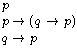This sequence constitutes a proof of if q then p from the premise p because: (a) it is a finite sequence of formulas ending in if q then p, (b) the first member of the sequence is a member of the set of premises, (c) the second member of the sequence is a logical axiom (this is an instance of the first axiom schema of sentential logic), and (d) the last member of the sequence follows from the first two by the rule Modus Ponens. So all of the conditions for claiming that there is a proof of if q then p from p are met.

Logical Theorem Schema: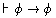This asserts that every instance of the schema is a theorem of logic. To prove this, consider the following sequence:This constitutes a proof of the schema from the empty set of premises because: (a) it is finite sequence of formulas ending with the schema, (b) line 1 is an instance of the second axiom schema of sentential logic, (b) line 2 is an instance of the first axiom schema of sentential logic, (c) line 3 follows from lines 1 and 2 by Modus Ponens, (d) line 4 is another instance of the first axiom schema, and (3) line 5 follows from lines 3 and 4 by Modus Ponens. As a particular example, it follows that if xF then xF is a theorem of logic.

## Metatheorems and Derived Rules

In what follows, we describe and sometimes prove rules of inference that can be derived from the basis of our logic. All of the natural deduction rules can be derived, though we only sketch a few of these rules.

Metatheorem (The Deduction Theorem) and Derived Rule (Conditional Proof)This claim constitutes a metatheorem about the system of Principia Metaphysica. It embodies the derived Rule of Conditional Proof. We shall not prove this metatheorem here, but rather, offer an example. Assume as a premise that All Greeks are European (let this be the sole element of the set of premises designated by the Greek letter Gamma in the statement of the metatheorem). Now consider the claim Clinton was Greek (let this be the designation of the Greek letter phi). It is a fact that by quantificational logic we can derive Clinton was European from the premise and claim (let Clinton was European be the designation of the Greek letter psi). And since Clinton was Greek has no free variables, the conditions of the metatheorem are met. So it tells us that from the premise All Greeks are European alone, we can derive If Clinton was Greek, Clinton was European. In what follows, when we prove a conditional (with an antecedent having no free variables) by assuming the antecedent and deriving the consequent, the justification for the proof is the Rule of Conditional Proof (CP).

Derived Rule: Reductio Ad Absurdum (RAA)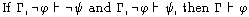To prove this is a good rule, we show that any proof of phi from Gamma involving RAA can be converted to a proof of phi from Gamma without using RAA. So suppose we have a proof of phi from Gamma using RAA. Then, by assumption, the antecedent of the rule is true. So if we apply the Deduction Theorem to the first conjunct of the rule's hypothesis, we know: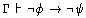Call a sequence of formulas that must exist according to this claim Sequence 1. Now if we apply the Deduction Theorem to the second conjunct of the rule's antecedent, we know:Again, call the sequence of formulas that must exist according to this claim Sequence 2. Now we produce a proof of phi from Gamma without using RAA as follows: combine Sequence 1 and Sequence 2; then append to this new sequence the third axiom of sentential logic (in "Logic/Sentential Logic"):;
now we append to the resulting sequence the result of applying Modus Ponens to the axiom just added and the last line of Sequence 1; finally we append to the resulting sequence the result of applying Modus Ponens to the last line and the last line of Sequence 2. The result constitutes a proof of phi from Gamma without using RAA.

Derived Rule: Modus TollensInstead of a proof, we offer an example: from the claims If the sun is shining, then John is happy and It is not the case that John is happy, it follows by Modus Tollens that It is not the case that the sun is shining. As a more specific example involving the language of the theory, it follows from the claims If it is possible that xF, then it is necessary that xF and It is not necessary that xF that It is not possible that xF.

Derived Rules: Double NegationFor example, from the claim John is happy it follows by Double Negation that It is not the case that it is not the case that John is happy, and vice versa. As a more specifc example involving the language of the theory, it follows from the claim that xF that It is not the case that it is not the case xF, and vice versa.

Derived Rule: & Introduction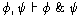For example, from the premises John is happy and Mary is sad we may draw the conjunctive conclusion John is happy & Mary is sad. Similarly, from aF and it is not the case that Fa, we may conclude aF & it is not the case that Fa.

Exercise: Find an introductory logic text and search for other rules of inference. Construct examples of these rules. Find rules for & Elimination, Disjunction Introduction and Elimination, Biconditional Introduction and Elimination, Contraposition and Disjunctive Syllogism.

## Some Further Theorems

We conclude with some examples that show the usefulness of the rules of Conditional Proof and RAA.

Logical Theorem Schema:To prove this Theorem Schema, first consider the following sequence of formulas: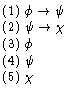Notice that this sequence of formulas demonstrates the following fact:The sequence demonstrates this fact because: (a) it is finite in length, (b) lines 1, 2, and 3 are all premises, and (c) lines 4 and 5 follow from previous lines by Modus Ponens. So, given this fact, it follows by the Deduction Theorem that:And given two further applications of the Deduction Theorem, we have proved:Exercise:Prove the Law of Excluded Middle and the Law of Noncontradiction using RAA.